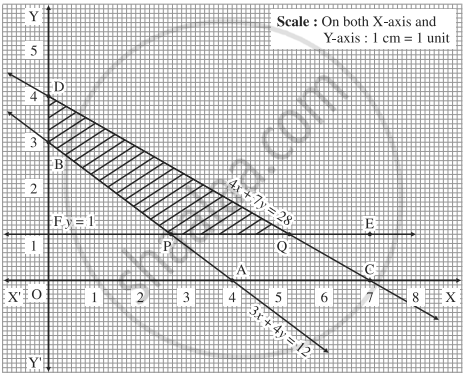Advertisement Remove all ads

# Find the feasible solution of the following inequation: 3x + 4y ≥ 12, 4x + 7y ≤ 28, y ≥ 1, x ≥ 0. - Mathematics and Statistics

Graph
Sum

Find the feasible solution of the following inequation:

3x + 4y ≥ 12, 4x + 7y ≤ 28, y ≥ 1, x ≥ 0.

Advertisement Remove all ads

#### Solution

First we draw the lines AB, CD and EF whose equations are 3x + 4y = 12 and  4x + 7y = 28 and y= 1 respectively.

 Line Equation Points on the X-axis Points on the Y-axis Sign Region AB 3x + 4y = 12 A (4,0) B (0,3) ≥ non-origin side of line AB CB 4x + 7y = 28 C (7,0) D(0,4) ≤ origin side of line CD EF y = 1 - F(0,1) ≥ non-origin side of line EFThe feasible solution is PQDB which is shaded in the graph.

Concept: Linear Programming Problem (L.P.P.)
Is there an error in this question or solution?
Advertisement Remove all ads

#### APPEARS IN

Advertisement Remove all ads
Advertisement Remove all ads
Share
Notifications

View all notifications

Forgot password?